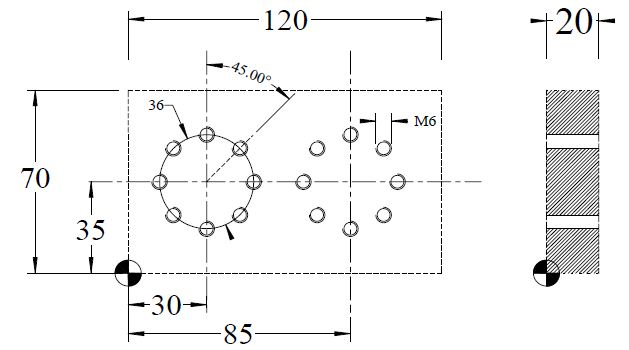# Circular Pattern (Cycle 220) Heidenhain Conversational Programming Exercise

Submit by: Brian

## Drawing/Image## CNC Program

```0 BEGIN PGM 10124 MM
1 BLK FORM 0.1 Z X+0 Y+0 Z-20
2 BLK FORM 0.2 X+120 Y+70 Z+0
3 TOOL DEF 1
4 TOOL DEF 2
5 TOOL DEF 3
6 TOOL CALL 1 Z S500
7 L M6
8 L M13
9 CYCL DEF 1.0 PECKING
10 CYCL DEF 1.1 SET UP 2
11 CYCL DEF 1.2 DEPTH -2
12 CYCL DEF 1.3 PECKG -2
13 CYCL DEF 1.4 DWELL 0
14 CYCL DEF 1.5 F200
15 CYCL DEF 220 POLAR PATTERN
Q216 = 30 ;CENTRE IN 1ST AXIS
Q217 = 35 ;CENTER IN 2ND AXIS
Q244 = 36 ;PITCH CIRCLE DIAMETR
Q245 = 0 ;STARTING ANGLE
Q246 = 360 ;STOPPING ANGLE
Q247 = 0 ;STEPPING ANGLE
Q241 = 8 ;NR OF REPETITIONS
Q200 = 2 ;SET-UP CLEARANCE
Q203 = 0 ;SURFACE COORDINATE
Q204 = 100 ;2ND SET-UP CLEARANCE
16 CYCL DEF 220 POLAR PATTERN
Q216 = 85 ;CENTRE IN 1ST AXIS
Q217 = 35 ;CENTER IN 2ND AXIS
Q244 = 36 ;PITCH CIRCLE DIAMETR
Q245 = 0 ;STARTING ANGLE
Q246 = 360 ;STOPPING ANGLE
Q247 = 0 ;STEPPING ANGLE
Q241 = 8 ;NR OF REPETITIONS
Q200 = 2 ;SET-UP CLEARANCE
Q203 = 0 ;SURFACE COORDINATE
Q204 = 100 ;2ND SET-UP CLEARANCE
17 TOOL CALL 2 Z S1000
18 L M6
19 L M13
20 CYCL DEF 1.0 PECKING
21 CYCL DEF 1.1 SET UP 2
22 CYCL DEF 1.2 DEPTH -20
23 CYCL DEF 1.3 PECKG 10
24 CYCL DEF 1.4 DWELL 0
25 CYCL DEF 1.5 F200
26 CYCL DEF 220 POLAR PATTERN
Q216 = 30 ;CENTRE IN 1ST AXIS
Q217 = 35 ;CENTER IN 2ND AXIS
Q244 = 36 ;PITCH CIRCLE DIAMETR
Q245 = 0 ;STARTING ANGLE
Q246 = 360 ;STOPPING ANGLE
Q247 = 0 ;STEPPING ANGLE
Q241 = 8 ;NR OF REPETITIONS
Q200 = 2 ;SET-UP CLEARANCE
Q203 = 0 ;SURFACE COORDINATE
Q204 = 100 ;2ND SET-UP CLEARANCE
27 CYCL DEF 220 POLAR PATTERN
Q216 = 85 ;CENTRE IN 1ST AXIS
Q217 = 35 ;CENTER IN 2ND AXIS
Q244 = 36 ;PITCH CIRCLE DIAMETR
Q245 = 0 ;STARTING ANGLE
Q246 = 360 ;STOPPING ANGLE
Q247 = 0 ;STEPPING ANGLE
Q241 = 8 ;NR OF REPETITIONS
Q200 = 2 ;SET-UP CLEARANCE
Q203 = 0 ;SURFACE COORDINATE
Q204 = 100 ;2ND SET-UP CLEARANCE
28 TOOL CALL 3 Z S500
29 L M6
30 L M13
33 CYCL DEF 2.0 TAPPING
34 CYCL DEF 2.1 SET UP 2
35 CYCL DEF 2.2 DEPTH -20
36 CYCL DEF 2.3 DWELL 0
37 CYCL DEF 2.4 F475
32 CYCL DEF 220 POLAR PATTERN
Q216 = 30 ;CENTRE IN 1ST AXIS
Q217 = 35 ;CENTER IN 2ND AXIS
Q244 = 36 ;PITCH CIRCLE DIAMETR
Q245 = 0 ;STARTING ANGLE
Q246 = 360 ;STOPPING ANGLE
Q247 = 0 ;STEPPING ANGLE
Q241 = 8 ;NR OF REPETITIONS
Q200 = 2 ;SET-UP CLEARANCE
Q203 = 0 ;SURFACE COORDINATE
Q204 = 100 ;2ND SET-UP CLEARANCE
33 CYCL DEF 220 POLAR PATTERN
Q216 = 85 ;CENTRE IN 1ST AXIS
Q217 = 35 ;CENTER IN 2ND AXIS
Q244 = 36 ;PITCH CIRCLE DIAMETR
Q245 = 0 ;STARTING ANGLE
Q246 = 360 ;STOPPING ANGLE
Q247 = 0 ;STEPPING ANGLE
Q241 = 8 ;NR OF REPETITIONS
Q200 = 2 ;SET-UP CLEARANCE
Q203 = 0 ;SURFACE COORDINATE
Q204 = 100 ;2ND SET-UP CLEARANCE
34 TOOL CALL 0
35 L M6
36 L M30
37 END PGM 10124 MM```